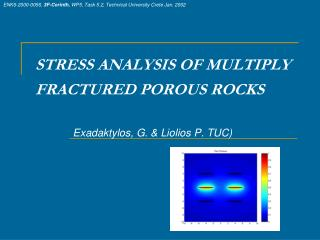DownloadDownload PresentationSTRESS ANALYSIS OF MULTIPLY FRACTURED POROUS ROCKS

# STRESS ANALYSIS OF MULTIPLY FRACTURED POROUS ROCKS

Télécharger la présentation## STRESS ANALYSIS OF MULTIPLY FRACTURED POROUS ROCKS

- - - - - - - - - - - - - - - - - - - - - - - - - - - E N D - - - - - - - - - - - - - - - - - - - - - - - - - - -
##### Presentation Transcript

1. ENK6-2000-0056, 3F-Corinth, WP5, Task 5.2, Technical University Crete Jan. 2002 STRESS ANALYSIS OF MULTIPLY FRACTURED POROUS ROCKS Exadaktylos, G. & Liolios P. TUC)

2. Introduction • A class of important geomechanical problems involves the quantification of critical conditions for crack initiation in the vicinity of singularities and stress concentrators in the geomaterial. This class of problems may be extended if the diffusion of pore pressure and/or heat is further considered.

3. Governing Equations of plane strain linear Elasticity

4. Simplification of the governing equations in short term and steady state states • Steady state This problem requires the solution for the total stresses (essentially the bi-harmonic eqn) and pore pressure (harmonic) which are uncoupled

5. Formulation of the two problems with the complex variable technique (isotropy)

6. Formulation of the two problems with the complex variable technique (anisotropy)

7. Boundary Conditions (i.e. 2nd kind for the elasticity problem and Dirichlet for pore pressure)

8. Cauchy integral representation of the solution of stresses and pore pressure

9. Formulation of the system of S.I.E’s

10. Numerical Integration of the system of singular integral equations of the 1st kind • Separation of density into singular and regular part

11. Gauss-Chebyshev integration scheme for the stresses

12. Gauss-Chebyshev integration scheme for the pore pressure

13. Transformation of the integration and collocation points to cracks of arbitrary shape Linear transformation from the [-1,1] space to the length s of the curvilinear crack Liolios, P. and Exadaktylos, G. (2005), A solution of steady-state fluid flow in multiply fractured isotropic porous media, Int. J. Solids Structures, in print.

14. Convergence of the integration scheme for the pore pressure

15. Comparison of the integration scheme with the analytic solution for the stress (30 int. points)

16. Example 1: Parallel cracks under uniform pore pressure

17. Example 2: Multiple (40) cracks ahead of a horizontal crack under uniform pore pressure (steady state)

18. Simplification of the governing equations in short term and steady state states • Short term (Undrained Conditions) Hence, the solution of only the compatibility equation for the total stresses gives the full short term solution of the problem

19. Example 3 – Inclined open crack in uniaxial compression and undrained conditions

20. Conclusions - Recommendations • A fast solver has been developed for multiply fractured media that takes into account pore pressure, stresses and temperature in steady state. • The consideration of time and crack propagation will be performed in a next step. • Interaction of holes, thin inclusions, cracks in anisotropic media will be also included in the numerical code.